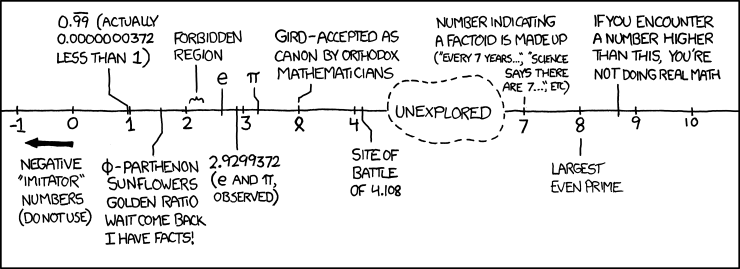# Largest Known Prime Number

We have a new largest known prime number! So here is an obligatory post about prime numbers.

In 2008, GIMPS (Great Internet Mersenne Prime Search) found the prime number $$2^{43112609}-1$$, with just under 13 million digits this was the largest known prime number. Well yesterday this record was smashed with the discovery of $$2^{57885161}-1$$ with 17,425,170 digits.

So what is a prime? If you are reading this post, I’m sure you already know but it never hurts to go over things you already know. Most people will say that a prime number is a number which is only divisible by itself and 1. This is an “okay” definition, but isn’t quite right. For example this does not explain why 1 is not a prime number, or, the fact that a prime number is of course also divisible by -1 (which is neither itself or 1!). Perhaps a slightly better definition could be:

Definition: A number is prime if:

• it only has two positive factors, and
• it is not 1.

Note here that we include, by definition, that 1 is not prime. There are a number of reasons for 1 not being a prime number, but the main reason I would say is for the Fundamental Theorem of Arithmetic, which deserves a post all to itself which I’ll write at another time. Switching on my undergrad brain there is another, more broad, definition of a prime which I would like to share with you:

Definition: A non-zero, non-unit element p in a ring R is prime if for all $$a,b\in R$$, $p|ab \Rightarrow p|a\mbox{ or } p|b.$

So, there you go :p. That clears that up…

For the not technically trained among us that might look rather confusing, it isn’t, trust me. The only ‘extra’ bit of maths you need is to know what a ring is.

Definition: The ordered tripe $$(R,+,\cdot)$$ where $$R$$ is a set and $$+,\cdot$$ are binary operations is a ring if and only if:

1. It is associative with respect to (w.r.t.) + (i.e. $$a+(b+c)=(a+b)+c$$)
2. It is commutative w.r.t. + (i.e. $$a+b=b+a$$)
3. There is an additive identity (i.e. there exists a number, lets represent it by $$o$$, such that $$a+o=o+a=a$$ basically, there is a ‘zero’)
4. There is an additive inverse (i.e. there is a number which takes another number back to the zero, $$a+a’=a’+a=o$$)
5. It is associative w.r.t. $$\cdot$$ (i.e. $$a\cdot (b\cdot c)=(a\cdot b)\cdot c$$)
6. Multiplication is distributive over addition: $a\cdot (b+c)=a\cdot b+a\cdot c,$ $(a+b)\cdot c=a\cdot c +b\cdot c.$

This defines structures that you already know (and love!) for example the set of all integers, $$\ldots,-3,-2,-1,0,1,2,3,\ldots$$ with the operations of addition and multiplication is a ring. We can very easily check that, by stepping through the 6 points of the definition above. The first two are clear (even if you didn’t realise that this isn’t always true!) for example you always knew that $$1+2=2+1$$. The third and fourth are also obvious, the number zero matches the requirements of point three, i.e. $$5+0=0+5=5$$, and the negative of any number gives us point 4, i.e. $$4+(-4)=(-4)+4=0$$. Point five – trivial – again, you know $$3\times 2=2\times 3$$. Finally point six, is something you know to be true, but perhaps will take a little longer to convince yourself.

So back to our definition of a prime number, now we know what a ring is, the only other bit of info we need to understand the more complicated looking definition is what the symbol $$|$$ means, i.e. what $$p|ab$$ means. The vertical line means ‘is a factor of’ so $$p|ab$$ means $$p$$ is  a factor of $$ab$$, where $$ab$$ are two numbers multiplied together.

Therefore in our definition of prime numbers the line, $p|ab \Rightarrow p|a\mbox{ or }p|b.$ Means if $$p$$ is a factor of the composite number $$ab$$ then $$p$$ is a factor of either $$a$$ or $$b$$.

This has taken me off the subject I had planned to write about, and this post is already quite long so I think I will wrap it up with simply a definition of what a Mersenne Prime is, which, if you can remember that long ago, is what we called this new very large prime number.

Mersenne prime’s (named after Marin Mersenne) are prime numbers of the form $$M_p=2^p-1$$. So the first few Mersenne primes are, $$M_2=2^2-1=3$$, $$M_3=2^3-1=7$$ and $$M_7=2^7-1=127$$.

The reason that we find very large prime numbers of this form is that there are a good number of fast algorithms for finding these primes, and indeed most of the largest prime numbers are Mersenne primes. Finding prime numbers of this size takes an unbelievable amount of computer hours, it took 39 days straight to check that this particular number was prime (which then had to be double, and triple, checked!). So imagine taking that long over each number you come across, you soon realise why it’s taken 5 years to find a larger prime number than the last one! (Remember there are an infinite number of primes, so they’ll always be a bigger one – although it has not been proven that there are an infinite number of Mersenne primes).

Well, that post didn’t really turn out how I expected, it turned into more of a definition fest, sorry about that, perhaps I’ll try and do a whole series of posts on Number Theory, so we can get beyond simple definitions. We’ll end with an xkcd comic to lighten the mood:xkcd 899: Number Line

This entry was posted in Mathematics and tagged , , , . Bookmark the permalink.

This site uses Akismet to reduce spam. Learn how your comment data is processed.